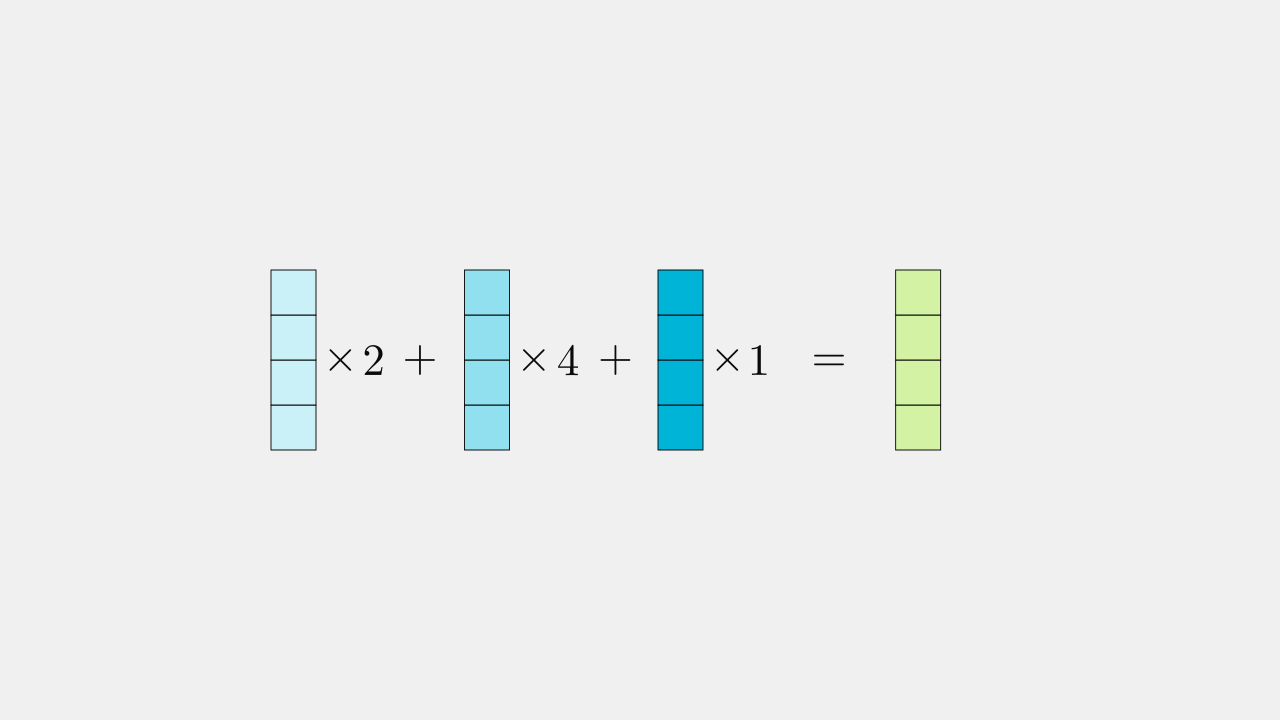# Visualizing Matrix Multiplication

Whenever I come across a matrix multiplication, my first attempt at visualizing it is to view the multiplication as:

multiple objects, combined together, many times

Matrices are usually carrying a list of objects, with each object represented by a row or column of the matrix. Inspecting how matrices behave by looking these objects can be an effective way to understand what an author is trying to communicate when they use matrices.

## Multiple objects, combined together

Matrix multiplication involves repeating a core operation. A single run of this operation goes like so:

The above operation can be described in words:

1. The input is 3 blue objects. The objects each have 4 elements.
2. Multiply each object by a number.
3. Sum together the results of the individual multiplications.
4. The sum is the output. It is another multi-element object with the same shape as the input objects.

The operation from the previous animation represents the following equation:This can be transformed into matrix notation:

To carry out this transition to matrix notation, we organize everything into columns of a matrix. The two matrices on the right have only one column.

The matrix notation excels at being compact, but it is not good at leveraging our visual intuition. For example, the notation alone doesn't reveal the relationships between the shapes of the matrices.

## Inputs and outputs have the same shape

While objects can have any number of elements, the operation of multiplication-summation preserves the shape of the objects, so the output object always looks like the input objects. Below we vary the shape of the input objects and the output object changes accordingly.

## Repeat the operation

The last thing to understand is the emergence of the second dimension of the output matrix. Matrix multiplication allows the core operation of multiplication-summation to be repeated an arbitrary number of times. Each time, we collect the result in a separate output object. Below, the operation is run a total of 9 times.

Below is the same 9 operations using matrix notation:

Using matrix notation, each new column of multiplication factors carries out a multiplication-summation operation. We can continue to add as many columns of multiplication factors as we wish, each one producing a corresponding output column in the matrix on the right hand side.

When the input objects are lists of numbers instead of lists of cubes we arrive at the standard syntax for a matrix product:

Without the colored columns the bare matrix syntax is void of almost any visual clues that explain the behavior or semantics of the matrix product.

## Why is matrix notation not more intuitive?

A justification for matrix notation might be the constraints of the 2D page and the desire to be concise. Another more forgivable reason is that matrix products are flexible enough to be interpreted in many different ways, and the standard syntax has been chosen to avoid giving preference to any interpretation. This flexibility means extra work on behalf of a reader: when thinking about matrix multiplication in terms of multiple objects, combined together, many times, matrix notation is flexible enough that the objects can be aligned as either columns or rows.

## Rows, columns, symmetry

There is a magical duality whereby the input objects can switch roles with the multiplication factors. More can be said about this, but for now, lets just examine the second way of using the matrix product to represent the concept of multiple objects, combined together, many times.

In the previous example we saw columns combined by columns. Below we see rows combining rows.

Multiplication factors appear as a row on the right followed by the input arranged as a list of rows. The output matches the input and is also a row. If we have many operations, the list of of multiplication factors grows downwards for each new operation.

## Recognizing the author's intention

It is quite often the case that if you come across a matrix product then the concept of combining multi-element objects is indeed a suitable interpretation. However, even if this interpretation is used, the symmetric nature of matrix multiplication means that the reader must figure out if the objects being combined are arranged as rows or columns.

While many interpretations of a matrix product are valid, depending on the context, one interpretation in particular often leads to more insight into what is being explained by an author. Unfortunately, authors often avoid explaining their preferred interpretation of things as doing makes their point less general.